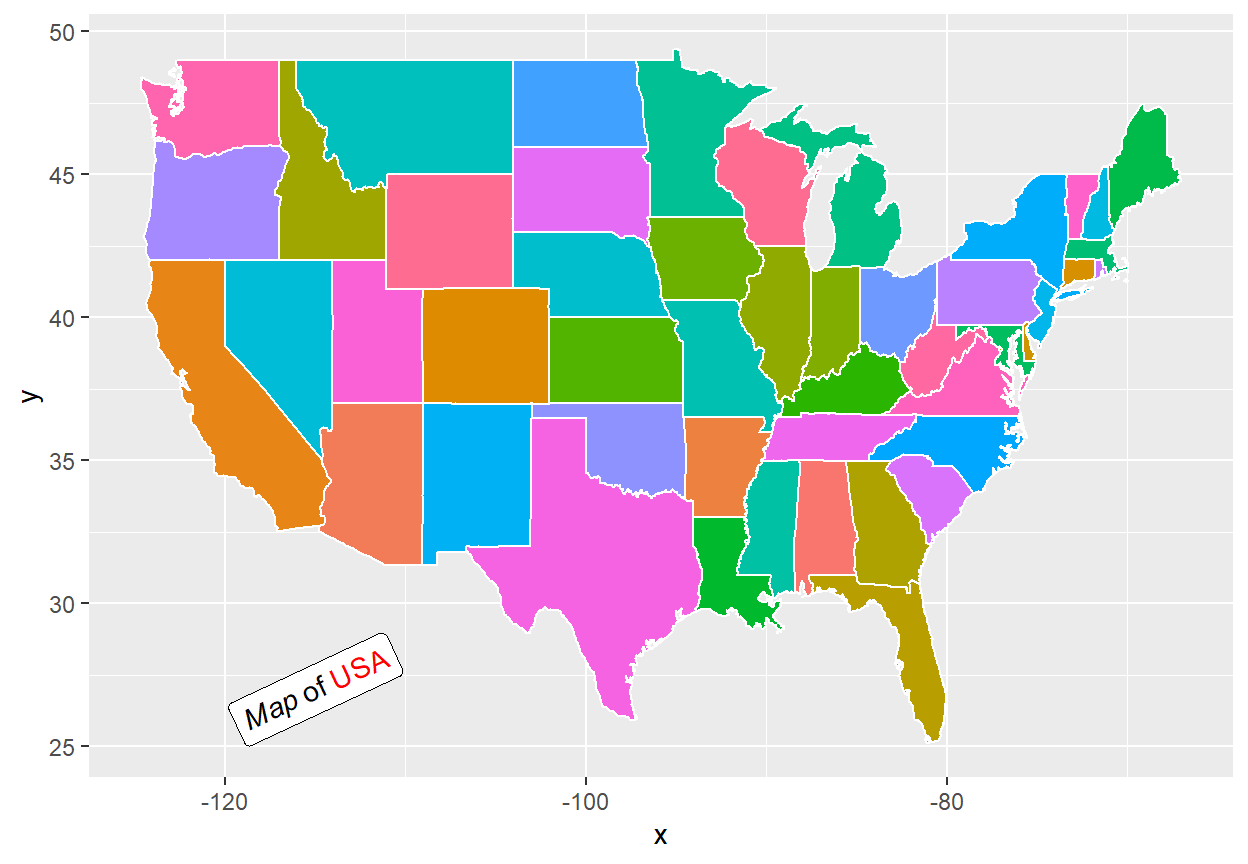# Text annotations in ggplot2

The `geom_text` and `geom_label` functions allows adding text or labels, respectively, to plots created with ggplot2. You can add some annotations to some coordinates or label data points.

``````# install.packages("ggplot2")
library(ggplot2)

# install.packages("maps")
library(maps)

df <- data.frame(x = state.center\$x, y = state.center\$y,
state = state.name)

p <- ggplot(df, aes(x = x, y = y)) +
geom_polygon(data = map_data("state"),
color = "white",
aes(x = long, y = lat,
fill = map_data("state")\$region,
group = group)) +
guides(fill = FALSE)
p``````

In this guide we are going to use the following example plot.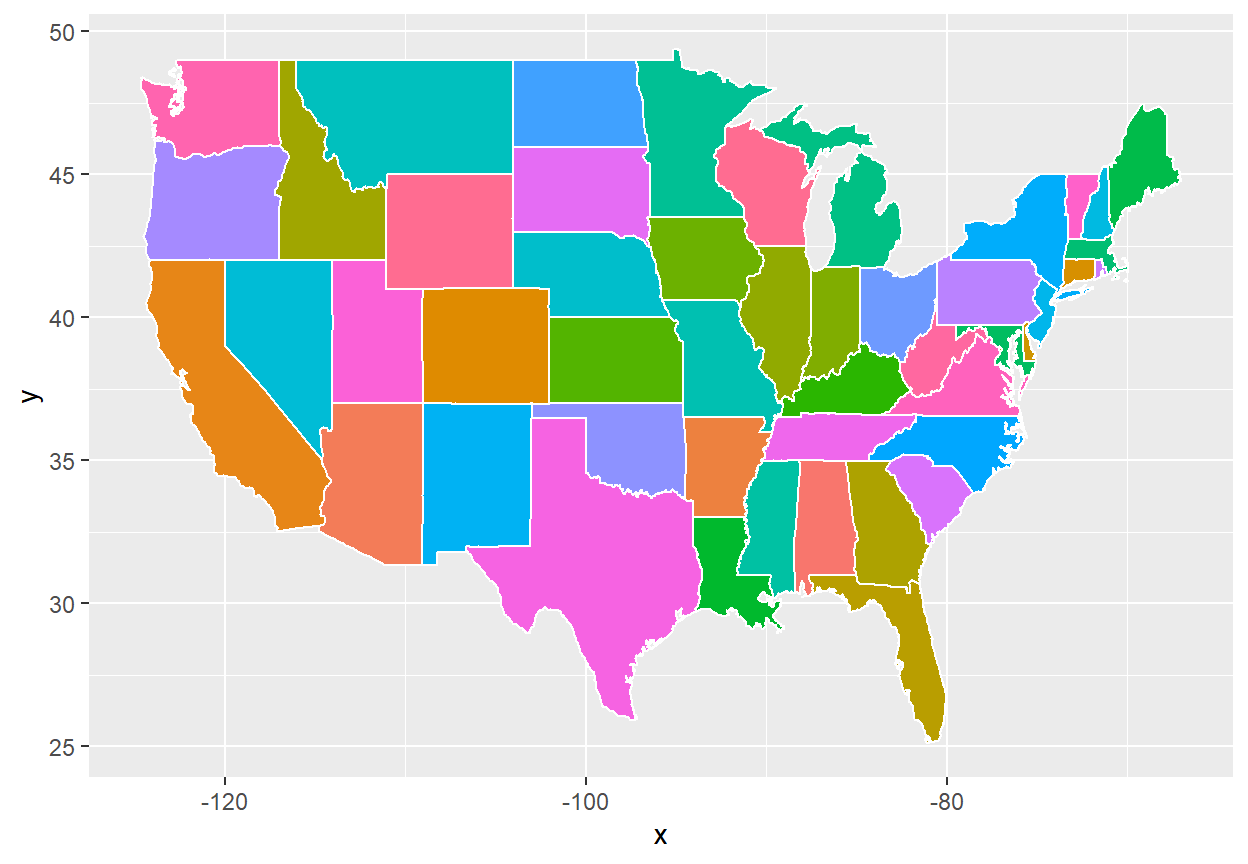## Adding text with `geom_text`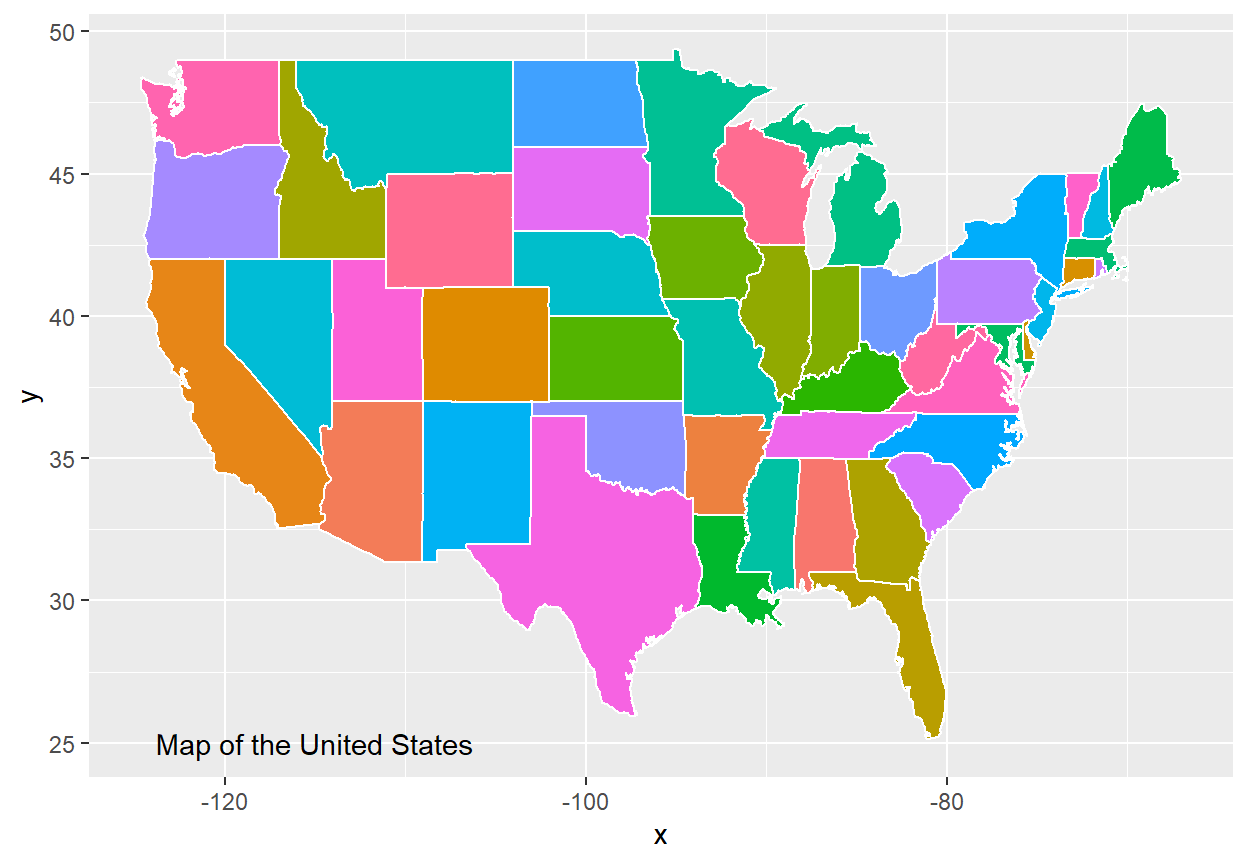``````p +
geom_text(aes(x = -115, y = 25,
label = "Map of the United States"),
stat = "unique")``````

Set `stat = "unique"`, otherwise the label will be redrawn for each data point on your data frame.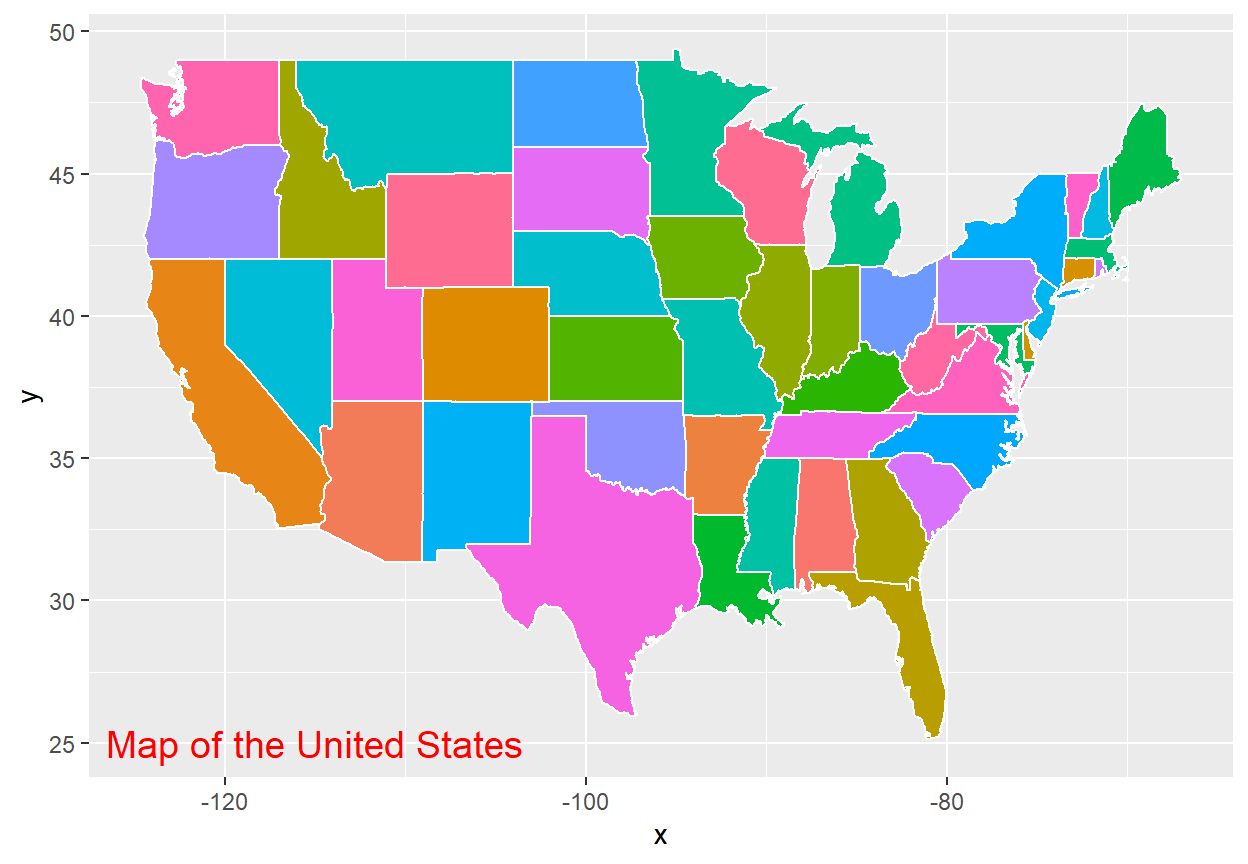Customizing the annotations

There are several arguments that you can customize, such as the color or the size of the text.

``````p +
geom_text(aes(x = -115, y = 25,
label = "Map of the United States"),
stat = "unique",
size = 5, color = "red")``````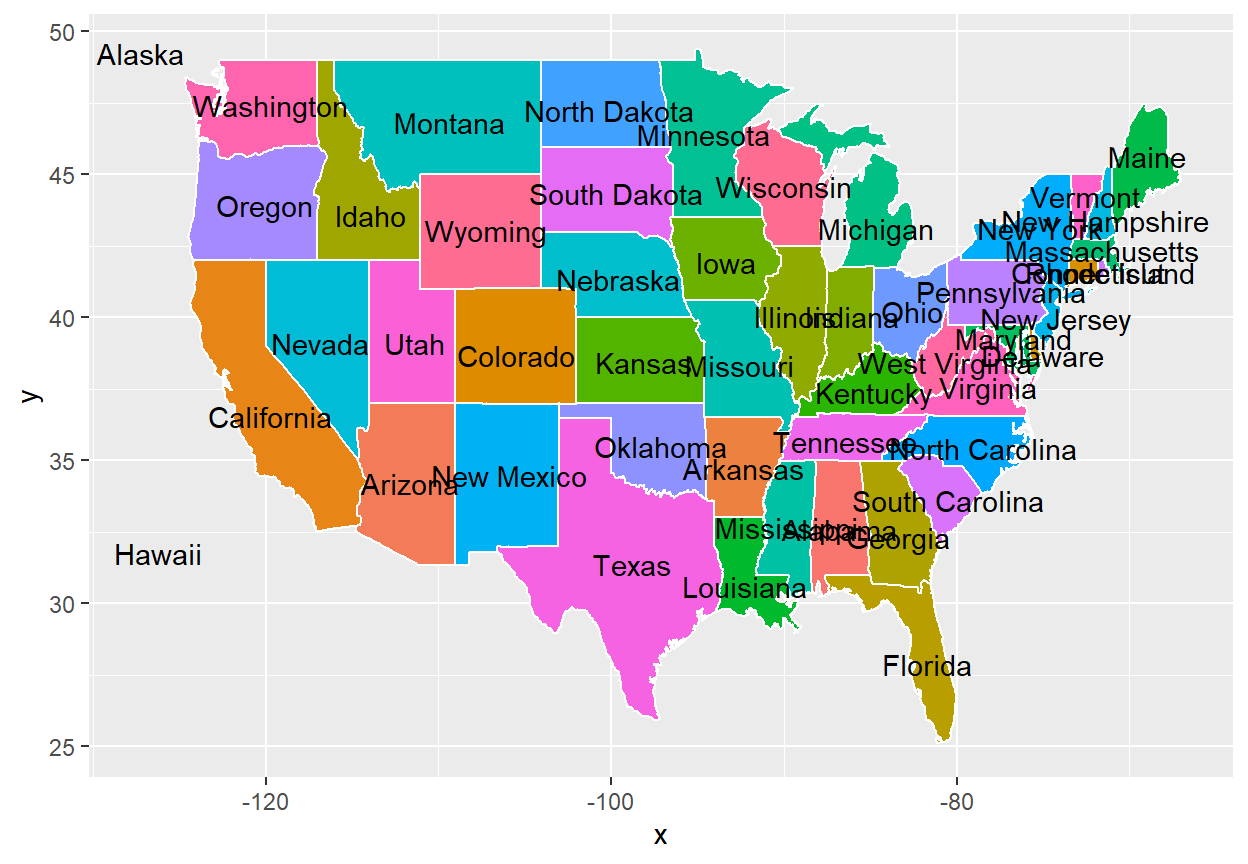If your data set contains a variable with groups or labels you can pass it to the `label` argument.

``````p +
geom_text(aes(label = state))``````

## Adding labels with `geom_label`

If you prefer adding labels instead of raw text use `geom_label`. The function behaves the same as the previous but with a background, making the text easier to read.

Label annotation

``````p +
geom_label(aes(x = -115, y = 25,
label = "Map of the United States"),
stat = "unique")``````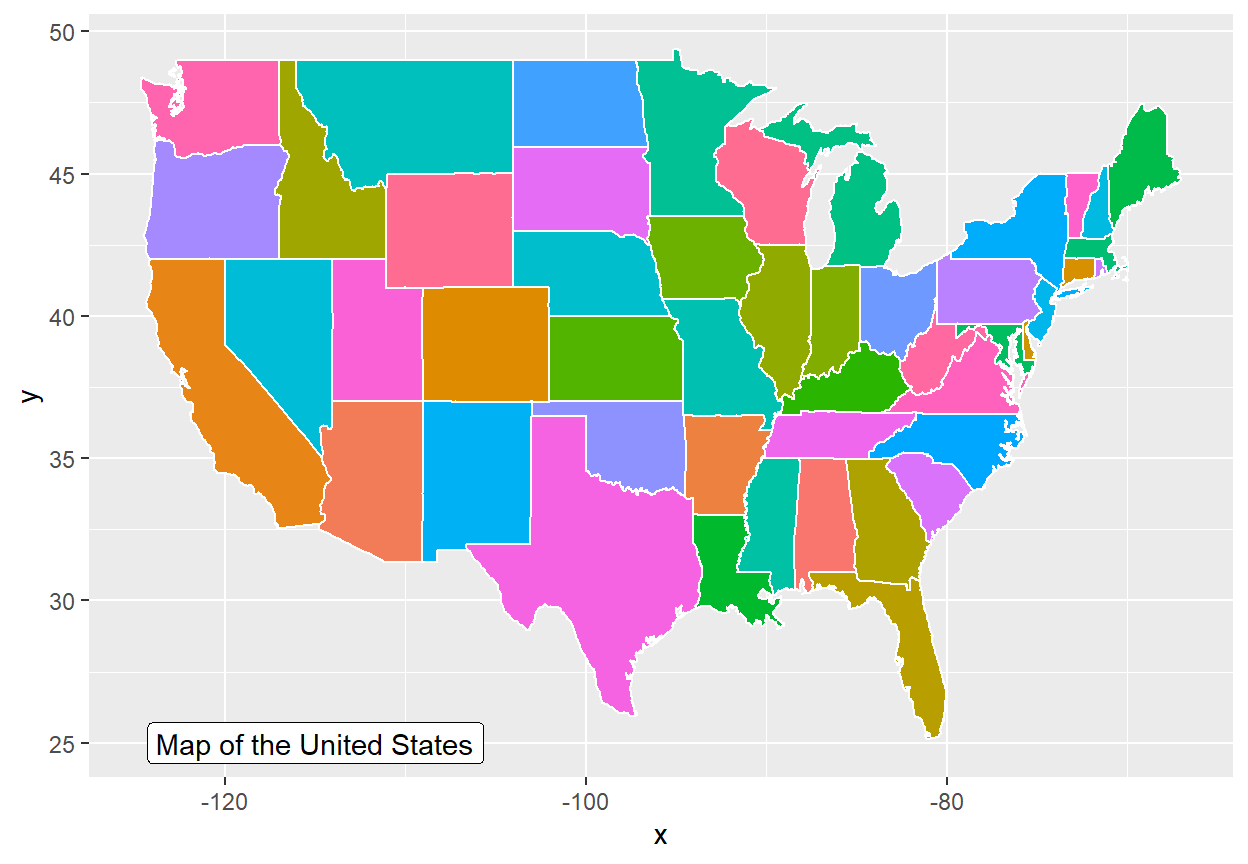Customizing the label

``````p +
geom_label(aes(x = -115, y = 25,
label = "Map of the United States"),
stat = "unique",
size = 5, color = "red", fill = "green")``````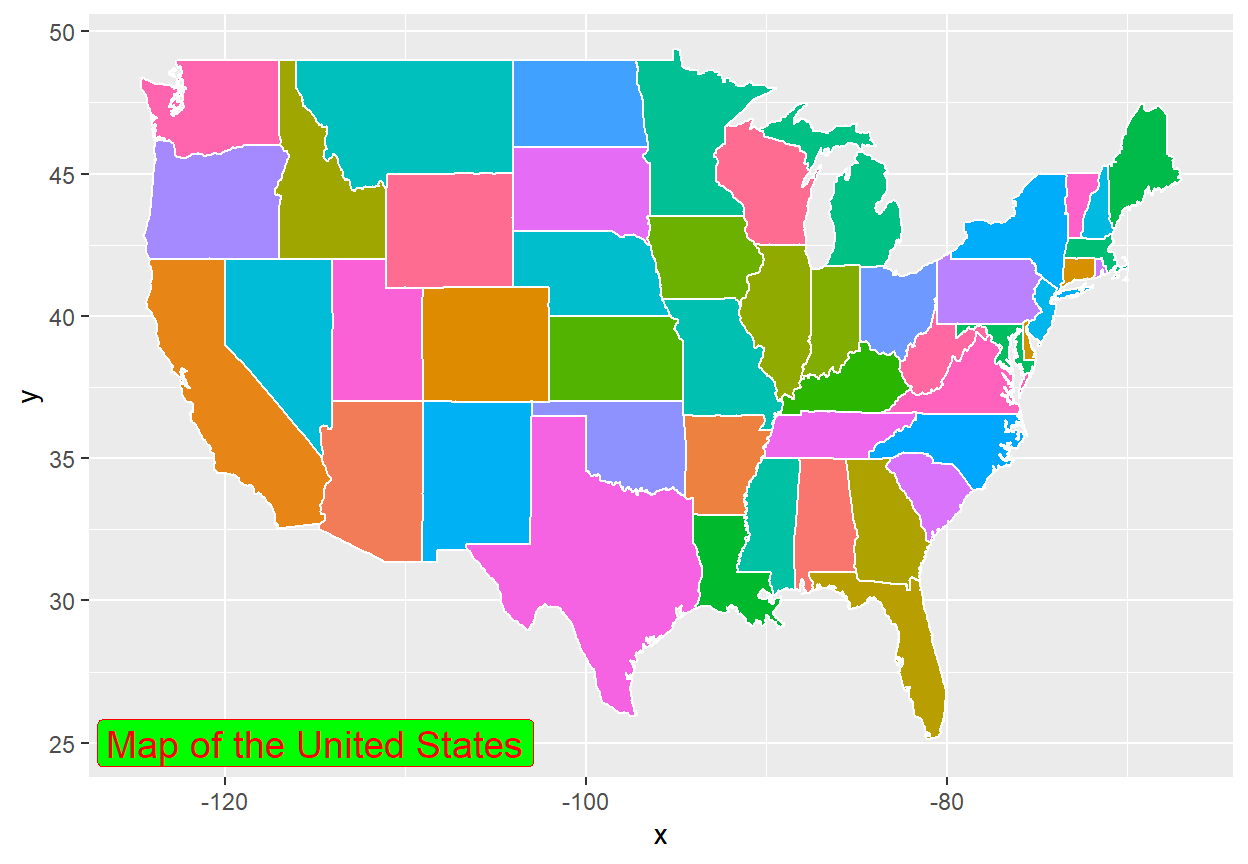Labelling points

``````p +
geom_label(aes(label = state))``````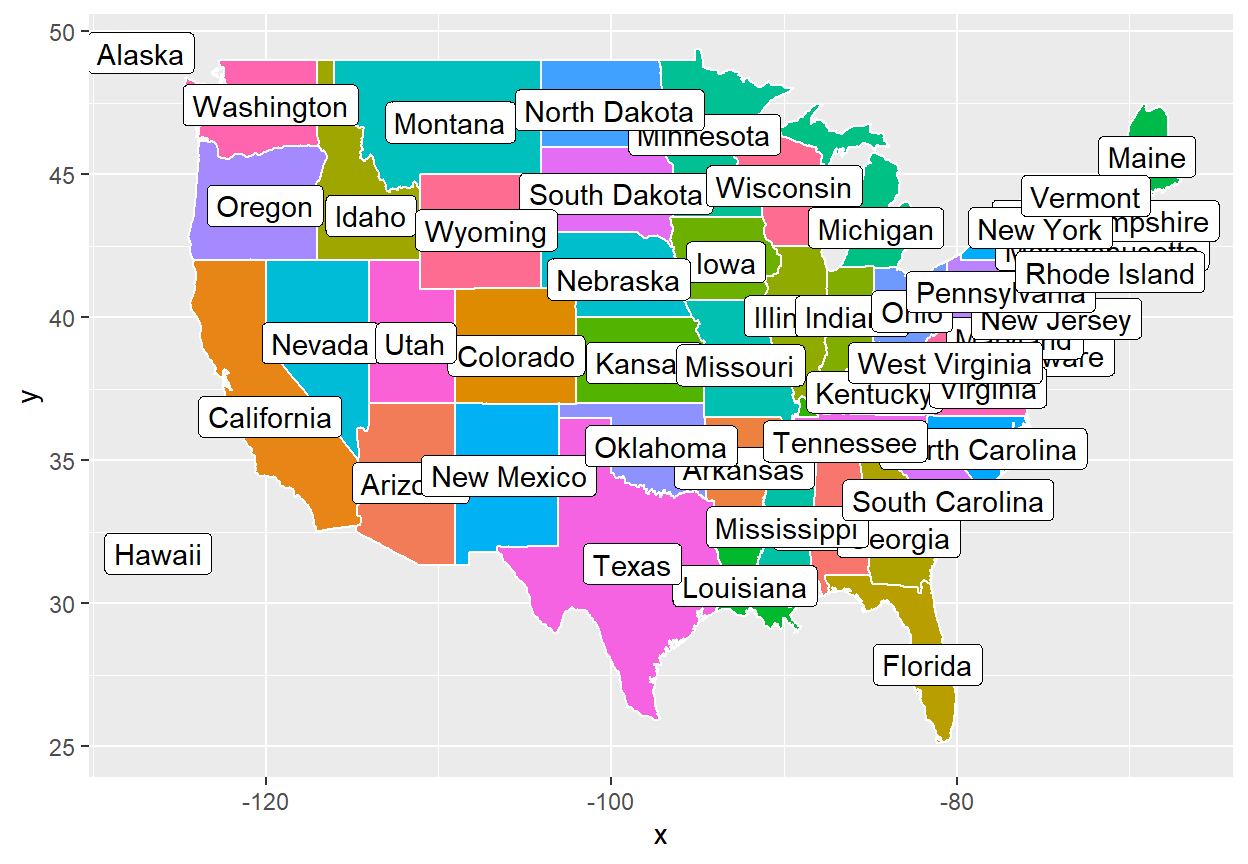Use `ggrepel` to avoid overlapping of the texts or labels.

## Avoid overlapping with `ggrepel`

The text and the labels are placed on the coordinates you set, but can overlap. The `ggrepel` package provides `geom_text_repel` and `geom_label_repel` functions, which make the labels repel away from each other as much as possible.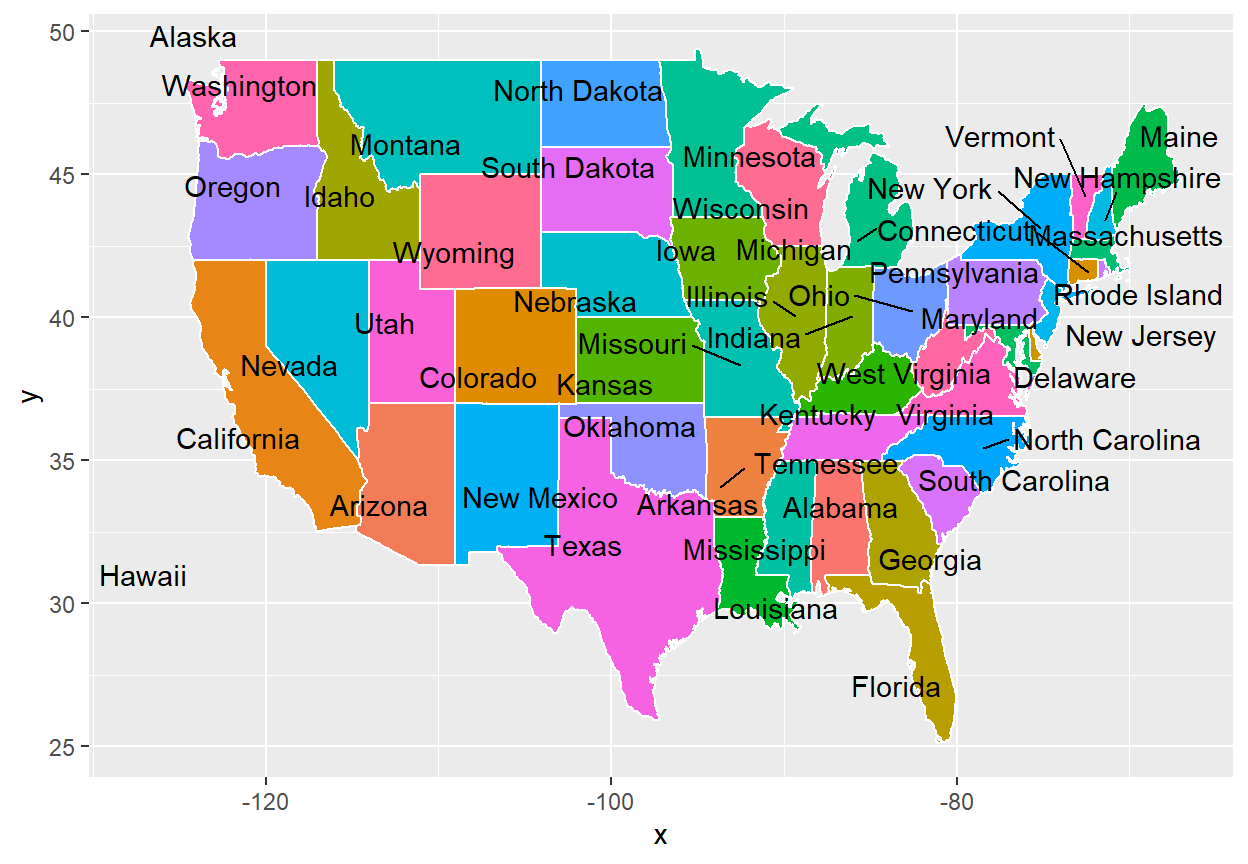geom_text_repel

``````# install.packages("ggrepel")
library(ggrepel)

p +
geom_text_repel(aes(label = state))``````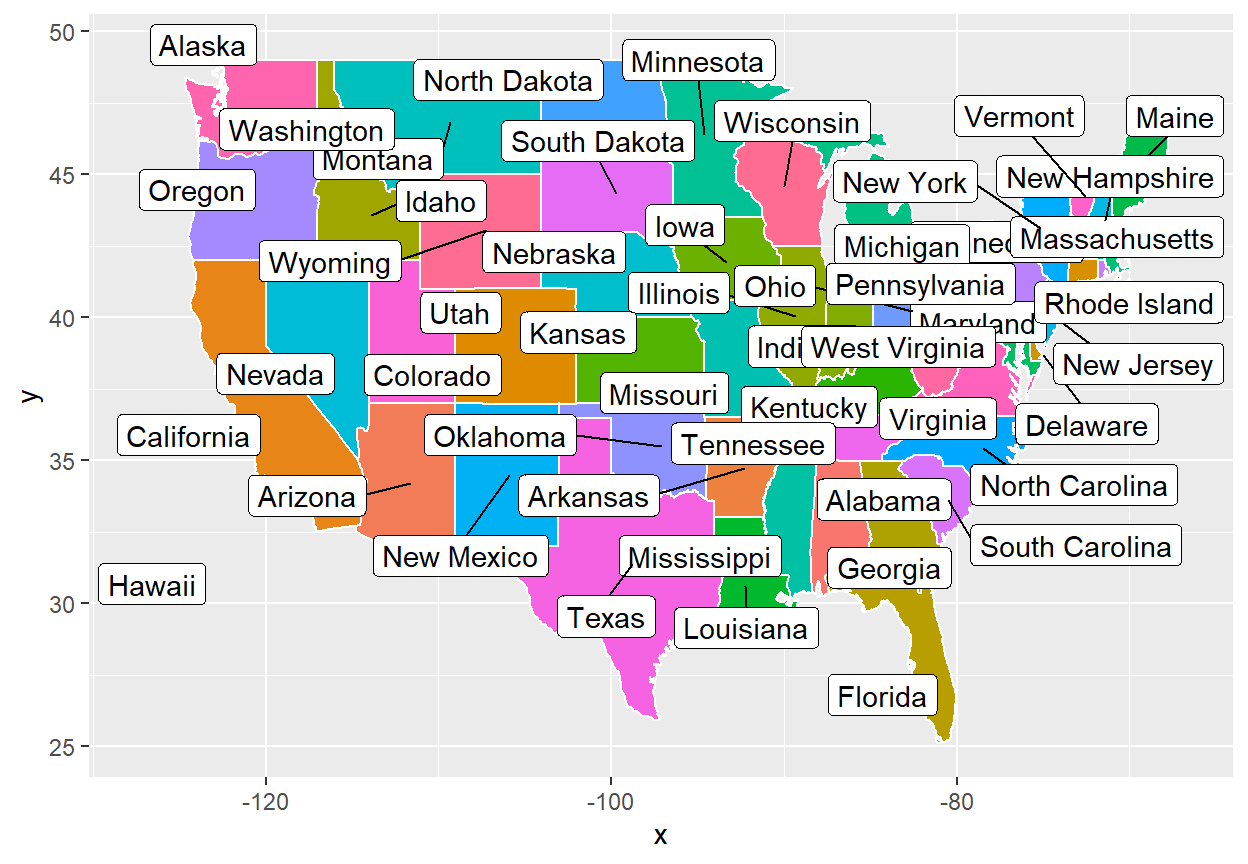geom_label_repel

``````# install.packages("ggrepel")
library(ggrepel)

p +
geom_label_repel(aes(label = state))``````

You can customize the colors, fonts and other arguments the same way as with `geom_text` or `geom_label`. See the package examples for more use cases.

## Use markdown and HTML with `ggtext`

If you want to fully customize you annotations use the `geom_richtext` function from `ggtext`, which allows you to add markdown and HTML formatting to your text annotations.

``````# install.packages("ggtext")
library(ggtext)

lab <- "*Map* of <span style = 'color:red'>USA</span>"
p +
geom_richtext(aes(x = -115, y = 25,
label = lab))``````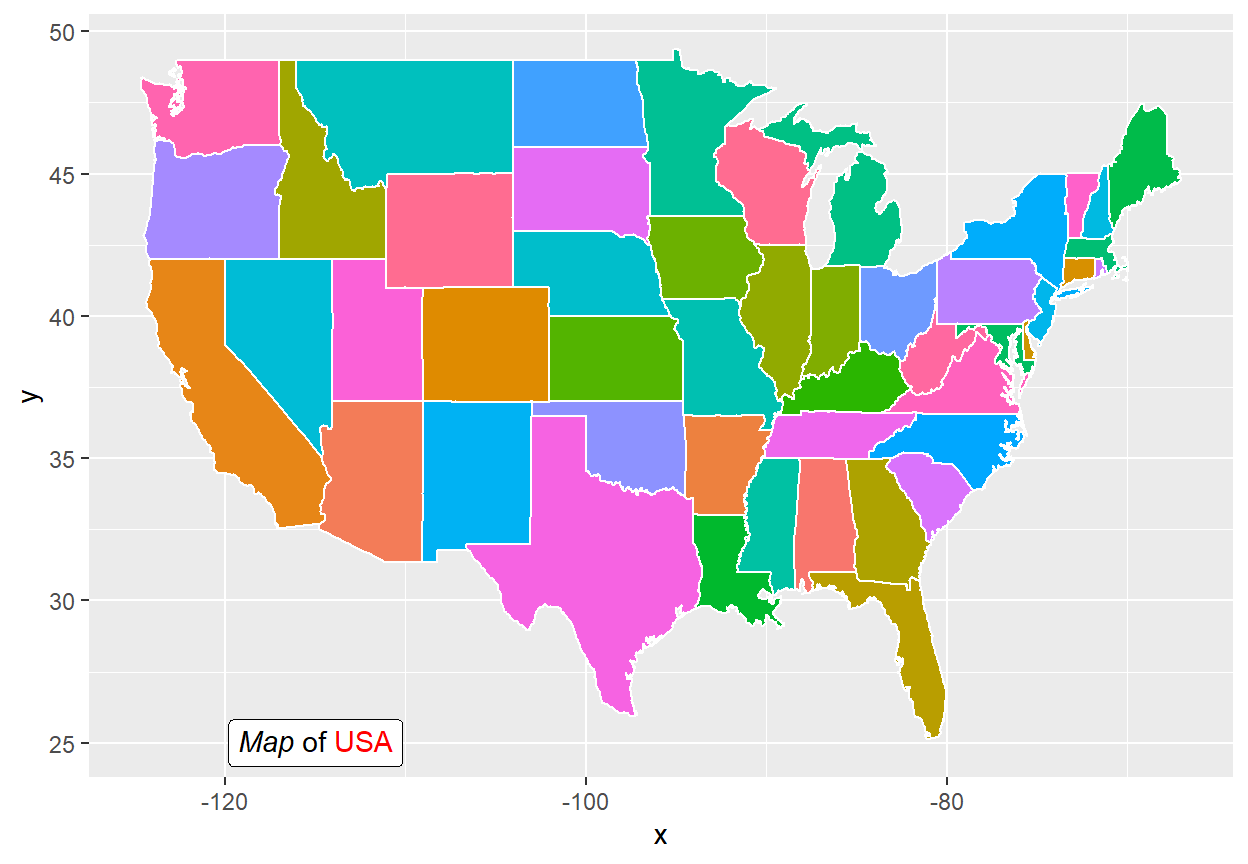Rotate the text

You can even rotate the text, a feature that is not available with default ggplot2.

``````# install.packages("ggtext")
library(ggtext)

lab <- "*Map* of <span style = 'color:red'>USA</span>"
p +
geom_richtext(aes(x = -115, y = 27,
label = lab),
angle = 25)``````